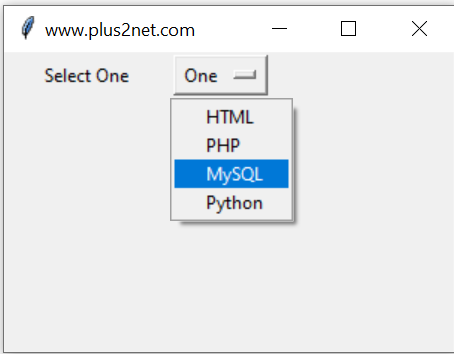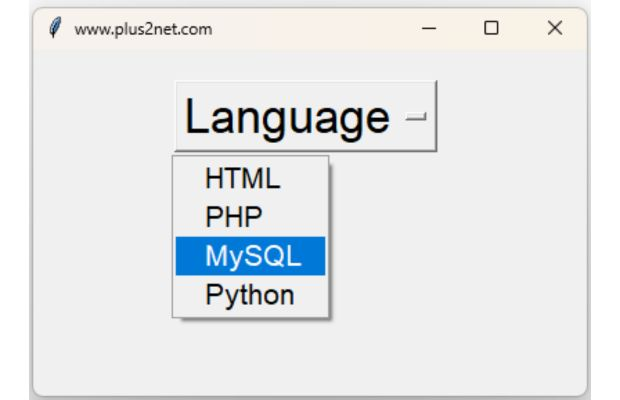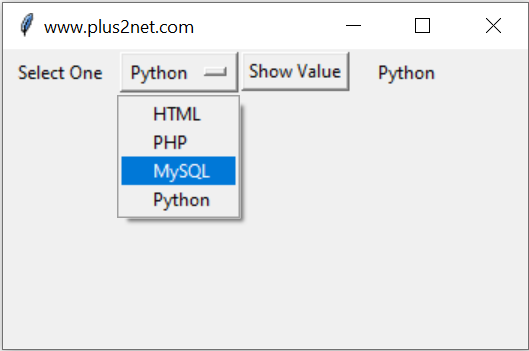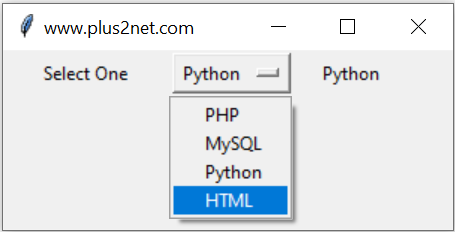OptionMenu is similar to dropdown list box where user can expand and select one of the several available options.
Tkinter OptionMenu to show dropdown list box with options from List and dictionary values to users

Using basics of Tkinter we will create one OptionMenu. (above Image )
``````import tkinter as tk
my_w = tk.Tk()
my_w.geometry("300x200")  # Size of the window

options = tk.StringVar(my_w)
options.set("One") # default selected value

l3 = tk.Label(my_w,  text='Select One', width=15 )
l3.grid(row=5,column=1)

om1 =tk.OptionMenu(my_w, options, "HTML","PHP", "MySQL", "Python")
om1.grid(row=5,column=2)
my_w.mainloop()  # Keep the window open``````
We can use elements of an list as options of a OptionMenu. Here we used `*my_list` to unpack the list return the elements as options. You can try the command `print(*my_list)` to display the elements of any list.
``````my_list = ["PHP","MySQL","Python","HTML"]
options = tk.StringVar(my_w)
options.set(my_list) # default value

om1.grid(row=5,column=2)``````

## Using different font family and size for the menu & options``````import tkinter as tk
my_w = tk.Tk()
my_w.geometry("400x250")  # Size of the window

options = tk.StringVar(value='Language')

my_w.mainloop()  # Keep the window open``````

options.get() , here options is the textvariable of OptionMenu.

Tkinter OptionMenu reading selected option & updating label on click of button, trace of StringVarWe will use one Button and on Click of the Button we will display the selected option of an OptionMenu inside another Label.

To the above code we will add one more Button and one Label to display the selected value of the OptionMenu. We used the function my_show() to execute once the Button is clicked.
``b1 = tk.Button(my_w,  text='Show Value',command=lambda: my_show())``
Inside the function my_show() we will read the value of Tkinter StringVar() options ( which is the textvariable of OptionMenu opt1 ) and display the same through our Label l2 textvariable str_out.
``````def my_show():
str_out.set(options.get())``````
The full code is here.
``````import tkinter as tk
my_w = tk.Tk()
my_w.geometry("350x200")  # Size of the window

options = tk.StringVar(my_w)
options.set("One") # default value

l1 = tk.Label(my_w,  text='Select One', width=10 )
l1.grid(row=2,column=1)

om1 =tk.OptionMenu(my_w, options, "HTML","PHP", "MySQL", "Python")
om1.grid(row=2,column=2)

b1 = tk.Button(my_w,  text='Show Value', command=lambda: my_show() )
b1.grid(row=2,column=3)

str_out=tk.StringVar(my_w)
str_out.set("Output")

l2 = tk.Label(my_w,  textvariable=str_out, width=10 )
l2.grid(row=2,column=4)

def my_show():
str_out.set(options.get())

my_w.mainloop()``````

## On Select or On Change of option of OptionMenuWithout using any Button click we can read the selected value of the OptionMenu. Whenever any selection is changed or one option is selected by the user we will read the value and display the same through a Label.
Here the variable options is connected to the OptionMenu, this value whenever changes ( when user select or change the option ) the trace method will trigger the callback function my_show().

Inside this function my_show() we will update the variable str_out ( which is connected to output Label l2) by reading the new assigned value of options i.e options.get().
``````def my_show(*args):
str_out.set(options.get())

options.trace('w',my_show)``````
Full code is here
``````import tkinter as tk
my_w = tk.Tk()
my_w.geometry("300x120")  # Size of the window

l3 = tk.Label(my_w,  text='Select One', width=15 )
l3.grid(row=2,column=1)

my_list = ["PHP","MySQL","Python","HTML"]
options = tk.StringVar(my_w)
options.set(my_list) # default value

om1.grid(row=2,column=2)

str_out=tk.StringVar(my_w)
str_out.set("Output")

l2 = tk.Label(my_w,  textvariable=str_out, width=10 )
l2.grid(row=2,column=4)

def my_show(*args):
str_out.set(options.get())

options.trace('w',my_show)
my_w.mainloop()  # Keep the window open``````

We have seen HTML drop down list box were the option attribute is collected but different value is shown to the visitor. So far we have used one single element list only to populate the OptionMenu here. Now we will use key value pairs or dictionary as options for the OptionMenu. Here is one sample dictionary from Category table where we have cat_id and category value as dictionary key , value pair.
``````import tkinter as tk
my_w = tk.Tk()
my_w.geometry("250x200")  # Size of the window
# create the dictionary
my_dict1={1: 'Fruits', 2: 'Colors', 3: 'Games', 4: 'Vehicles'}

options = tk.StringVar(my_w) # variable
options.set(my_dict1) # default value

om1.grid(row=2,column=5)

def my_show(*args):  # on select function
for i,j in my_dict1.items():
if j==options.get():
print(i)

options.trace('w',my_show)
my_w.mainloop()``````
Here Dictionary category ( value ) is displayed but on selection ID ( key ) is collected and displayed.

Subscribe to our YouTube Channel here

## Subscribe

* indicates required
Subscribe to plus2netplus2net.com